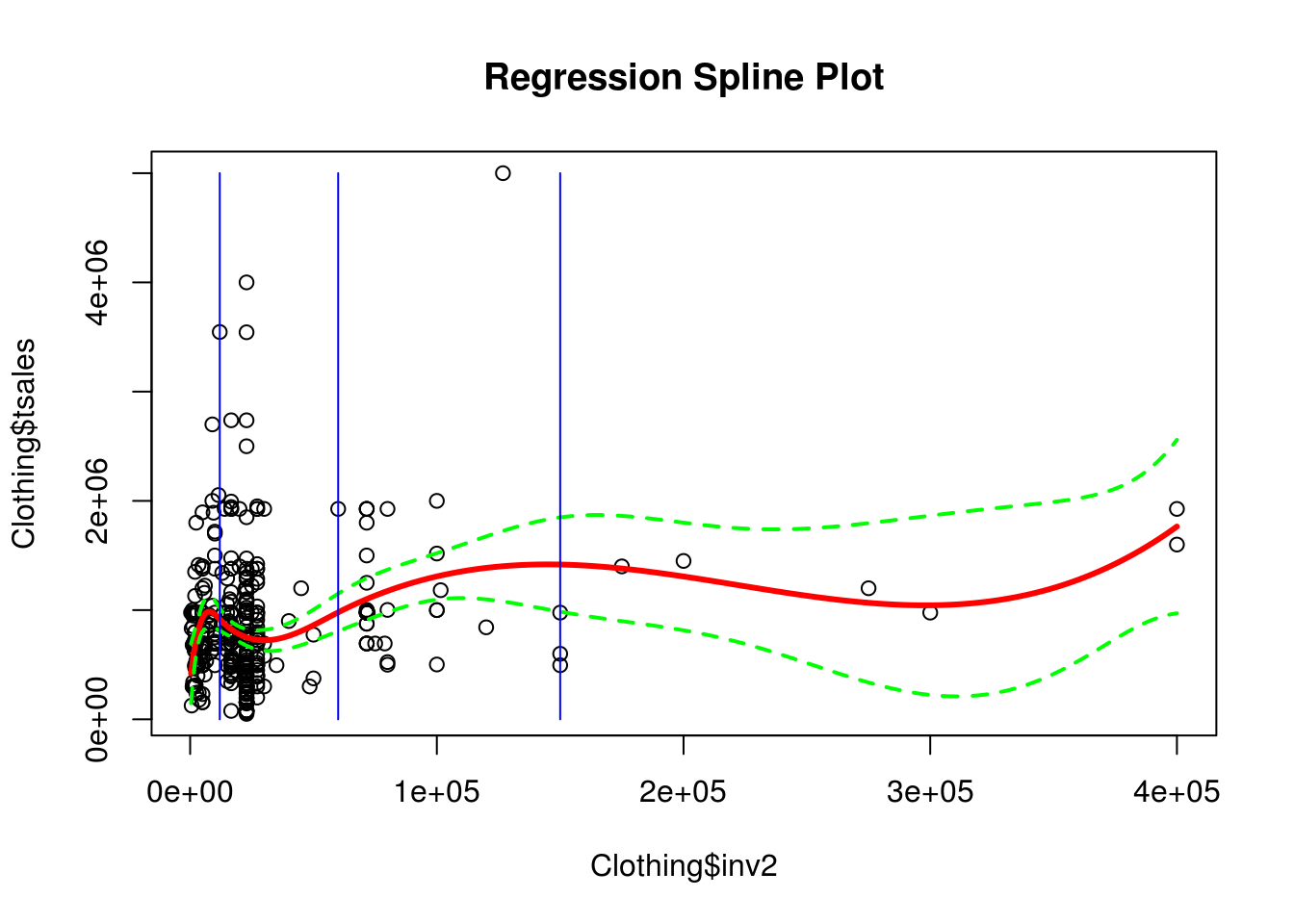# Polynomial Spline Regression in R

Normally, when least squares regression is used, you fit one line to the model. However, sometimes you may want enough flexibility that you fit different lines over different regions of your independent variable. This process of fitting different lines over different regions of X is known as Regression Splines.How this works is that there are different coefficient values based on the regions of X. As the researcher, you can set the cutoff points for each region. The cutoff point is called a “knot.” The more knots you use the more flexible the model becomes because there are fewer data points with each range allowing for more variability.

We will now go through an example of polynomial regression splines. Remeber that polynomial means that we will have a curved line as we are using higher order polynomials. Our goal will be to predict total sales based on the amount of innovation a store employs. We will use the “Ecdat” package and the “Clothing” dataset. In addition, we will need the “splines” package. The code is as follows.

``library(splines);library(Ecdat)``
``data(Clothing)``

We will now fit our model. We must indicate the number and placement of the knots. This is commonly down at the 25th 50th and 75th percentile. Below is the code

``fit<-lm(tsales~bs(inv2,knots = c(12000,60000,150000)),data = Clothing)``

In the code above we used the traditional “lm” function to set the model. However, we also used the “bs” function which allows us to create our spline regression model. The argument “knots” was set to have three different values. Lastly, the dataset was indicated.

Remember that the default spline model in R is a third-degree polynomial. This is because it is hard for the eye to detect the discontinuity at the knots.

We now need X values that we can use for prediction purposes. In the code below we first find the range of the “inv2” variable. We then create a grid that includes all the possible values of “inv2” in increments of 1. Lastly, we use the “predict” function to develop the prediction model. We set the “se” argument to true as we will need this information. The code is below.

``````inv2lims<-range(Clothing\$inv2)
inv2.grid<-seq(from=inv2lims,to=inv2lims)
pred<-predict(fit,newdata=list(inv2=inv2.grid),se=T)``````

We are now ready to plot our model. The code below graphs the model and includes the regression line (red), confidence interval (green), as well as the location of each knot (blue)

``````plot(Clothing\$inv2,Clothing\$tsales,main="Regression Spline Plot")
lines(inv2.grid,pred\$fit,col='red',lwd=3)
lines(inv2.grid,pred\$fit+2*pred\$se.fit,lty="dashed",lwd=2,col='green')
lines(inv2.grid,pred\$fit-2*pred\$se.fit,lty="dashed",lwd=2,col='green')
segments(12000,0,x1=12000,y1=5000000,col='blue' )
segments(60000,0,x1=60000,y1=5000000,col='blue' )
segments(150000,0,x1=150000,y1=5000000,col='blue' )``````When this model was created it was essentially three models connected. Model on goes from the first blue line to the second. Model 2 goes form the second blue line to the third and model three was from the third blue line until the end. This kind of flexibility is valuable in understanding  nonlinear relationship